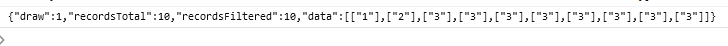# DataTable always return error Requested unknown parameter even if json is correct

but column 'NamaKategori' IS returned just fine in console log, I don't understand where is my problem here.

here is the console log of my AJAXhere is my Javascript Code:

``````\$('#example').DataTable( {
processing: true,
serverSide: true,
ajax: {
url: 'lib/kategori_list.php',
type: 'POST'
},
"columns": [
{ "data": "NamaKategori" }
],
dom: 'Brt',
buttons: [
'copy', 'csv', 'excel', 'pdf', 'print'
]
} );
``````

and here is my "kategori_list.php"

``````include_once('../koneksi/koneksi.php');

include_once('akses_get.php');

// Table name and primary key
\$table = 'kategori';
\$primaryKey = 'IDKategori';

// Columns to include in the result set
\$columns = array(
array( 'db' => 'NamaKategori', 'dt' => 0 )
);

// Build the SQL query
\$sql = "SELECT NamaKategori FROM \$table";

// Apply filtering, if any
if (!empty(\$_POST['search']['value'])) {
\$search = \$_POST['search']['value'];
\$sql .= " WHERE NamaKategori LIKE '%\$search%' OR Catatan LIKE '%\$search%'";
}

// Apply sorting, if any
if (!empty(\$_POST['order'])) {
\$orderBy = \$_POST['columns'][\$_POST['order']['column']]['data'];
\$orderDir = \$_POST['order']['dir'];
\$sql .= " ORDER BY \$orderBy \$orderDir";
}

// Apply paging, if any
if (\$_POST['start'] != 0 || \$_POST['length'] != -1) {
\$start = \$_POST['start'];
\$length = \$_POST['length'];

\$sql .= " LIMIT \$start, \$length";
}

// Execute the query
\$result = \$connection->query(\$sql);

// Build the response object
\$response = array(
"draw" => intval(\$_POST['draw']),
"recordsTotal" => \$result->num_rows,
"recordsFiltered" => \$result->num_rows,
"data" => array()
);

// Add each row of data to the response
while (\$row = \$result->fetch_assoc()) {
\$response['data'][] = array_values(\$row);
}

// Close the database connection
\$connection->close();

// Return the response as JSON
echo json_encode(\$response);
``````

• If you could like to a test case showing the issue, that would be really helpful. If not, can you use the debugger to give me a trace please - click the Upload button and then let me know what the debug code is.

Allan

• @allan, hello. thanks for the reply

I don't quite understand what this debugger about, But I have put the code in my web and click upload button.

• You need to give me the code that the debugger generates after the upload is complete. That way I can access the trace.

Allan

• @allan, sorry for the trouble.

I just create a new project and do a basic select until it working... then add the parameter one by one to exactly like my code above and it just works...

I don't understand why but I take that,

anyway, sorry for the hassle, thanks

• Yup - if it works, that's awesome! Glad to hear you've got it working!

Allan